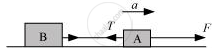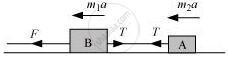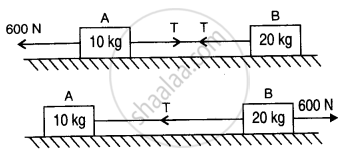CBSE (Science) Class 11CBSE
Share

# Two Bodies of Masses 10 Kg and 20 Kg Respectively Kept on a Smooth, Horizontal Surface Are Tied to the Ends of a Light String. a Horizontal Force F = 600 N is Applied to (I) A, (Ii) B Along the Direction of String. What is the Tension in the String in Each Case? - CBSE (Science) Class 11 - Physics

ConceptNewton’s Second Law of Motion

#### Question

Two bodies of masses 10 kg and 20 kg respectively kept on a smooth, horizontal surface are tied to the ends of a light string. A horizontal force F = 600 N is applied to (i) A, (ii) B along the direction of string. What is the tension in the string in each case?

#### Solution 1

Horizontal force, F = 600 N

Mass of body A, m1 = 10 kg

Mass of body B, m2 = 20 kg

Total mass of the system, m = m1 + m2 = 30 kg

Using Newton’s second law of motion, the acceleration (a) produced in the system can be calculated as:

F = ma

:.a = F/m = 600/30 = 20 "m/s"^2

When force F is applied on body A:The equation of motion can be written as:

F – T = m1a

T = F – m1a

= 600 – 10 × 20 = 400 N … (i)

When force F is applied on body B:The equation of motion can be written as:

F – T = m2a

T = F – m2a

∴T = 600 – 20 × 20 = 200 N … (ii)

#### Solution 2

Accleration = (600 N)/(10 kg + 20 kg) = 20 ms^(-2)

(i) When force is applied on 10 kg mass

600 - T  = 10 x 20 or

T = 400 N

(ii)When force is applied on 50 kg mass

600 - T = 20 x 20 or

T = 200 NIs there an error in this question or solution?

#### APPEARS IN

Solution Two Bodies of Masses 10 Kg and 20 Kg Respectively Kept on a Smooth, Horizontal Surface Are Tied to the Ends of a Light String. a Horizontal Force F = 600 N is Applied to (I) A, (Ii) B Along the Direction of String. What is the Tension in the String in Each Case? Concept: Newton’s Second Law of Motion.
S